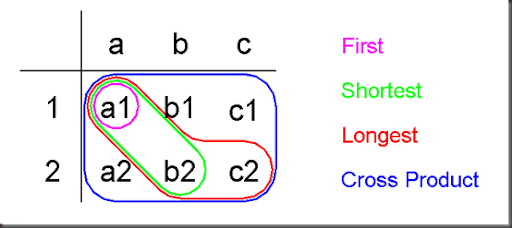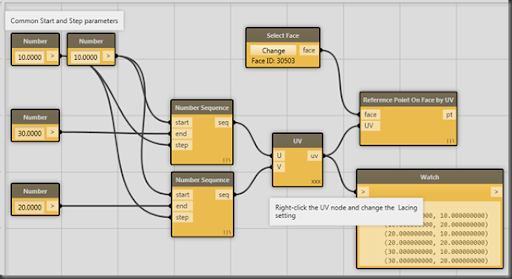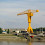## Tuesday, 2 July 2013

### Lacing

I wasn’t sure that I understood the new Lacing functionality in Dynamo (This post is based on Dynamo 0.5.0), so I did some experiments.

Each node in Dynamo has a Lacing setting (right-click the node, and select Lacing from the context menu), which can be First, Shortest, Longest, or Cross Product. Lacing controls how the node deals with list inputs. Each setting has a tooltip that gives you some guidance. For example, Shortest’s tooltip is: ‘For two lists {a,b,c}{1,2} returns {a1,b2}’

So the idea here is that you have a two-input node (a UV node for example), which can take a list on each input. The Lacing setting tells the node how to combine the elements from each list: Do you want all the combinations, or just a selection?

Based on the tooltips, I drew up this table:The two input lists {a, b, c} and {1, 2} are along the top and at the left. In the middle of the table are the possible combinations. (a1), (a2), etc. And I’ve highlighted the combinations that are returned by each Lacing setting.

For example, First just returns (a1), so the UV node would just give you one UV element. Shortest returns (a1) and (b2), a List of two UV elements. And so on.

Put another way, First just combines the first element from each input list. Shortest combines the corresponding elements (a and 1, b and 2, etc.) from each list, until the shortest list runs out of elements. Longest does the same, except that it carries on combining until the longest list runs out of elements, re-using the last element from shorter lists as necessary. Cross Product combines all the elements from all the lists.

That’s the theory. How does it work in practice? Here is a layout I built to test the two-input case:This layout creates a pair of number sequences, {10, 20, 30} and {10, 20}, and feeds them to a UV node. The output of the UV node is used to create RefPoints on a Face.

Changing the Lacing of the UV node does generate each of these sequences:

First: {(10, 10)}

Shortest: {(10, 10) (20, 20)}

Longest: {(10, 10) (20, 20) (30, 20)}

Cross Product: {(10, 10) (10, 20) (20, 10) (20, 20) (30, 10) (30, 20)}

And here are the matching screenshots:

Next, Lacing with 3 lists!

1.2.